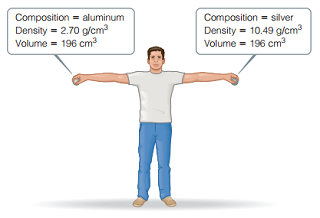# Problem: You may want to reference (Pages 15 - 16) Section 1.4 while completing this problem.Consider the two spheres shown here, one made of silver and the other of aluminum.The force of gravity acting on an object is F = mg, where m is the mass of an object and g is the acceleration of gravity (9.8 m/s2). How much work do you do on the silver sphere if you raise it from the floor to a height of 2.1 m?

###### FREE Expert Solution

We are asked how much work do you do on the silver sphere if you raise it from the floor to a height of 2.1 m.

94% (306 ratings)###### Problem Details

You may want to reference (Pages 15 - 16) Section 1.4 while completing this problem.

Consider the two spheres shown here, one made of silver and the other of aluminum.The force of gravity acting on an object is F = mg, where m is the mass of an object and g is the acceleration of gravity (9.8 m/s2). How much work do you do on the silver sphere if you raise it from the floor to a height of 2.1 m?